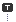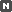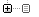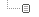Every unexpected event is a path to learning for you.

### 카테고리분류 전체보기 (2326)Unity3D (570)Programming (472)Unreal (4)Gamebryo (56)Tip & Tech (185)협업 (34)3DS Max (3)Game (12)Utility (116)Etc (92)Portfolio (19)Subject (90)iOS,OSX (38)Android (13)Linux (5)잉여 프로젝트 (2)게임이야기 (1)Memories (19)Interest (37)Thinking (36)한글 (26)PaperCraft (5)Animation (408)Wallpaper (2)재테크 (19)Exercise (3)나만의 맛집 (2)냥이 (9)육아 (9)
Total1,345,498
Today270
Yesterday332### 달력

« » 2019.12
1 2 3 4 5 6 7
8 9 10 11 12 13 14
15 16 17 18 19 20 21
22 23 24 25 26 27 28
29 30 31

### 'array'에 해당되는 글 4건

1. 2014.11.21 Sorting Arrays
2. 2012.10.24 Inspector에 다차원 배열 값 셋팅하기(for C#) (1)
3. 2011.05.09 Array -> String
4. 2010.11.26 Arrays in C++/CLI

## Sorting Arrays

Programming/C# / 2014. 11. 21. 11:53

# Sorting Arrays [C#]

This example shows how to sort arrays in C#. Array can be sorted using static method Array.Sortwhich internally use Quicksort algorithm.

## Sorting array of primitive types

To sort array of primitive types such as intdouble or string use method Array.Sort(Array) with the array as a paramater. The primitive types implements interface IComparable, which is internally used by the Sort method (it calls IComparable.Com­pareTo method). See example how to sort int array:

[C#]

```// sort int array
int[] intArray = new int { 8, 10, 2, 6, 3 };
Array.Sort(intArray);
// write array
foreach (int i in intArray) Console.Write(i + " ");  // output: 2 3 6 8 10

```

or how to sort string array:

[C#]

```// sort string array
string[] stringArray = new string { "X", "B", "Z", "Y", "A" };
Array.Sort(stringArray);
// write array
foreach (string str in stringArray) Console.Write(str + " "); // output: A B X Y Z

```

## Sorting array of custom type using delegate

To sort your own types or to sort by more sophisticated rules, you can use delegate to anonymous method. The generic delegate Comparison<T> is declared as `public delegate int Comparison<T> (T x, T y)`. It points to a method that compares two objects of the same type. It should return less then 0 when X < Y, zero when X = Y and greater then 0 when X > Y. The method (to which the delegate points) can be also an anonymous method (written inline).

Following example demonstrates how to sort an array of custom type using the delegate to anonynous comparison method. The custom type in this case is a class User with properties Name and Age.

[C#]

```// array of custom type
User[] users = new User { new User("Betty", 23),  // name, age
new User("Susan", 20),
new User("Lisa", 25) };

```

[C#]

```// sort array by name
Array.Sort(users, delegate(User user1, User user2) {
return user1.Name.CompareTo(user2.Name);
});
// write array (output: Betty23 Lisa25 Susan20)
foreach (User user in users) Console.Write(user.Name + user.Age + " ");

```

[C#]

```// sort array by age
Array.Sort(users, delegate(User user1, User user2) {
return user1.Age.CompareTo(user2.Age); // (user1.Age - user2.Age)
});
// write array (output: Susan20 Betty23 Lisa25)
foreach (User user in users) Console.Write(user.Name + user.Age + " ");

```

## Sorting array using IComparable

If you implement IComparable interface in your custom type, you can sort array easily like in the case of primitive types. The Sort method calls internally IComparable.Com­pareTo method.

[C#]

```// custom type
public class User : IComparable
{
// ...

// implement IComparable interface
public int CompareTo(object obj)
{
if (obj is User) {
return this.Name.CompareTo((obj as User).Name);  // compare user names
}
throw new ArgumentException("Object is not a User");
}
}

```

Use it as you sorted the primitive types in the previous examples.

[C#]

```// sort using IComparable implemented by User class
Array.Sort(users);  // sort array of User objects

```

#### 'Programming > C#' 카테고리의 다른 글

 [C#] 시간체크(Stopwatch)  (0) 2015.09.15 2015.02.12 2014.11.21 2014.09.29 2014.09.17 2014.09.16
TAG array, sort

## Inspector에 다차원 배열 값 셋팅하기(for C#)

Unity3D / 2012. 10. 24. 15:38

우선 그냥 임의로 한 방법이라..

더 좋은 방법이 있을진 나중에 알아보기로 하고..

1) 아래와 같은 다차원 배열에 사용 될 클래스를 하나 만든다.

```using UnityEngine;
using System.Collections;

[System.Serializable] //<== MonoBehaviour가 아닌 클래스에 대해 Inspector에 나타납니다.
public class Testitem
{
public int[] variable;    // 하위에 넣어질 배열 변수
}```

※ Serializable 참조 : http://docs.unity3d.com/Documentation/ScriptReference/Serializable.html

2) GameObject에 링크 된 스크립트에 아래와 같이 변수 선언을 한다.

```public Testitem[] arrayTestItem;
```

3) 그리고 해당 GameObject의 Inspector를 보면 'Array Test Item'이 보인다.

4) Size에 1이상의 숫자를 넣어보면 Element가 생기면서 화살표(▶)가 있는게 보인다.

5) 화살표(▶)를 눌러보면 1)에서 만든 Testitem의 변수인 variable이 추가돼 있는게 보인다.

6) 원하는 사이즈 넣고 입력..

7) 결과는 아래 스샷과 같음..#### 'Unity3D' 카테고리의 다른 글

 안드로이드 빌드 셋팅하기  (22) 2012.10.25 2012.10.25 2012.10.24 2012.10.24 2012.10.22 2012.10.19

### 댓글을 달아 주세요

1. 2018.07.08 01:25 ddd

사용은 어떻게 하는지 알려줘야 하는데 참 답답하다

## Array -> String

Programming/C# / 2011. 5. 9. 02:12

요새 c#으로 삽질중이다.

오늘은 배열로 한 한시간 가까이 날려 먹은거 같은데;;

Array라는 타입이 있다. 모든 배열의 기초 클래스란다

요넘을 String[]로 옮기고 싶은 거다...

즉...

Array Buffer = new Array;

이런 녀석이 있다면

String[] szBuffer = Buffer;

이 짓을 하고 싶다는 거지;;

한참을 해맸지만 결론은 간단했다.

String[] szBuffer = (String)Buffer;

허탈하네 - _-;;

[출처]
C#에서 배열|작성자 시네루진

#### 'Programming > C#' 카테고리의 다른 글

 C# 관련 정보 사이트[MKEXDEV.NET]  (0) 2011.09.13 2011.05.13 2011.05.09 2011.03.25 2011.03.23 2011.02.11
TAG array, string

## Arrays in C++/CLI

Programming/C++/CLI / 2010. 11. 26. 16:00
The article exposes the new array syntax available in C++/CLI for the declaration and use of CLI arrays

## Introduction

Managed arrays are allocated on the CLI heap as opposed to native arrays which are allocated on the unmanaged C++ heap, which essentially means that they are typical garbage collected .NET objects. And throughout this article the term array will refer to a managed array, and if at all a native array is mentioned, it will explicitly be termed as a native array. Some of you might have seen my article on using managed arrays with the old MC++ syntax and I am sure a few of you would have let out pained groans at the really peculiar syntax. Well, those people should be delighted to know that C++/CLI supports managed arrays using a far more intuitive syntax than which existed in the older syntax.

### Basic features

Here are some of the basic features of managed arrays in C++/CLI :-

• Syntax resembles that of C++ templates
• `System::Array` is automatic base type for all managed arrays
• Allocated on the CLR heap (means they get Garbage Collected)
• Rank of the array need not be 1 (arrays with rank 1 are called single dimensional and those with rank >1 are called multi dimensional)
• Easily supports jagged arrays (unlike in the older syntax, jagged arrays are easier to declare and use)
• Implicit conversion to and explicit conversion from `System::Array` BCL class
• The rank and dimensions of an array object cannot be changed, once an array has been instantiated

## Pseudo-template of an array type

The declaration and usage of array types in C++/CLI seems to use an imaginary template type (there is no such template of course, it�s all VC++ compiler magic) :-Collapse
```namespace stdcli::language
{
template<typename T, int rank = 1>
ref class array : System::Array {};
}```

`array` is declared inside the `stdcli::language` namespace so as to avoid conflicts with existing source code

## Single dimensional array usage

### Arrays of a reference typeCollapse
```ref class R
{
public:
void Test1(int x)
{
array<String^>^ strarray = gcnew array<String^>(x);
for(int i=0; i<x; i++)
strarray[i] = String::Concat("Number ",i.ToString());
for(int i=0; i<x; i++)
Console::WriteLine(strarray[i]);
}
};```

The syntax does look different from that used for native arrays; C++ coders who have used templates should find this a lot more intuitive than people who come from a non-C++ background, but eventually they'll get comfortable with it.

### Arrays of a value typeCollapse
```ref class R
{
public:
void Test2(int x)
{
array<int>^ strarray = gcnew array<int>(x);
for(int i=0; i<x; i++)
strarray[i] = i * 10;
for(int i=0; i<x; i++)
Console::WriteLine(strarray[i]);
}
};```

Unlike in the old syntax, array syntax for value types is exactly the same as that for managed types.

## Multi dimensional array usage

Multi dimensional arrays are managed arrays that have a rank greater than 1. They are not arrays of arrays (those are jagged arrays).Collapse
```ref class R
{
public:
void Test3()
{
array<String^,2>^ names = gcnew array<String^,2>(4,2);
names[0,0] = "John";
names[1,0] = "Tim";
names[2,0] = "Nancy";
names[3,0] = "Anitha";
for(int i=0; i<4; i++)
if(i%2==0)
names[i,1] = "Brown";
else
names[i,1] = "Wilson";
for(int i=0; i<4; i++)
Console::WriteLine("{0} {1}",names[i,0],names[i,1]);
}
};```

## Jagged arrays

Jagged arrays are non-rectangular, and are actually arrays of arrays. The new template-style array syntax simplifies the declaration and use of jagged arrays, which is a major improvement over the old syntax where jagged arrays had to be artificially simulated by the developer.Collapse
```ref class R
{
public:
void Test()
{
array<array<int>^>^ arr = gcnew array<array<int>^> (5);

for(int i=0, j=10; i<5; i++, j+=10)
{
arr[i] = gcnew array<int> (j);
}

Console::WriteLine("Rank = {0}; Length = {1}",
arr->Rank,arr->Length);
/*
Output :-
Rank = 1; Length = 5
*/

for(int i=0; i<5; i++)
Console::WriteLine("Rank = {0}; Length = {1}",
arr[i]->Rank,arr[i]->Length);
/*
Output :-
Rank = 1; Length = 10
Rank = 1; Length = 20
Rank = 1; Length = 30
Rank = 1; Length = 40
Rank = 1; Length = 50
*/
}
};```

### Using a `typedef` to simplify jagged array usageCollapse
```typedef array<array<int>^> JaggedInt32Array;
typedef array<array<String^>^> JaggedStringArray;

ref class R
{
public:
void Test()
{
JaggedInt32Array^ intarr = gcnew JaggedInt32Array(10);
JaggedStringArray^ strarr = gcnew JaggedStringArray(15);
}
};```

## Directly initialize an array

The new syntax allows painless direct initialization of arrays.Collapse
```ref class R1
{
public:
void Test()
{
//Single dimensional arrays

array<String^>^ arr1 = gcnew array<String^> {"Nish", "Colin"};
array<String^>^ arr2 = {"Nish", "Smitha"};

//Multi dimensional arrays

array<Object^,2> ^ multiobarr = {{"Nish", 100}, {"Jambo", 200}};
}
};```

## Arrays as function argumentsCollapse
```ref class R
{
public:
void ShowStringArray(array<String^>^ strarr)
{
for(int i=0; i<strarr->Length; i++)
Console::WriteLine(strarr[i]);
}
void Show2DInt32Array(array<int,2>^ arr)
{
for(int i=0; i<arr->GetLength(0); i++)
{
Console::WriteLine("{0} times 2 is {1}",arr[i,0],arr[i,1]);
}
}
};

void _tmain()
{
R^ r = gcnew R();
r->ShowStringArray(gcnew array<String^> {"hello", "world"});
array<int,2>^ arr = { {1,2}, {2,4}, {3,6}, {4,8} };
r->Show2DInt32Array(arr);
}

//Output :-

/*
hello
world
1 times 2 is 2
2 times 2 is 4
3 times 2 is 6
4 times 2 is 8
*/```

## Returning arrays from functionsCollapse
```ref class R
{
public:
array<String^>^ GetNames(int count)
{
array<String^>^ arr = gcnew array<String^>(count);
for(int i=0; i<count; i++)
{
arr[i] = String::Concat("Mr. ",(i+1).ToString());
}
return arr;
}

void ShowNames(array<String^>^ arr)
{
for(int i=0; i<arr->Length; i++)
Console::WriteLine(arr[i]);
}
};

void _tmain()
{
R^ r = gcnew R();
array<String^>^ arr = r->GetNames(7);
r->ShowNames(arr);
}```

## Array covariance

You can assign an array of type R to an array of type B, where B is a direct or indirect parent of R, and R is a `ref` class.Collapse
```ref class R1
{
//...

};

ref class R2 : R1
{
//...

};

void _tmain()
{
array<R1^>^ arr1 = gcnew array<R1^>(4);
array<R2^>^ arr2 = gcnew array<R2^>(4);

array<R1^>^ arr3 = arr2;
array<R1^>^ arr4 = gcnew array<R2^>(4);
}```

## Parameter arrays

C++/CLI has support for parameter arrays. There can only be one such parameter array per function and it also needs to be the last parameter.Collapse
```ref class R
{
public:
void Test(String^ s, [ParamArray] array<int>^ arr )
{
Console::Write(s);
for(int i=0; i<arr->Length; i++)
Console::Write(" {0}",arr[i]);
Console::WriteLine();
}
};

void _tmain()
{
R^ r = gcnew R();
r->Test("Nish");
r->Test("Nish",1);
r->Test("Nish",1,15);
r->Test("Nish",1,25,100);
}```

Right now the only supported syntax uses the `ParamArray` attribute, but the eventual syntax will also support the simpler style shown below :-

• `void Test(String^ s, ... array<int>^ arr )`

## Calling `System::Array` methods

Since every C++/CLI array is implicitly a `System::Array` object, we can use `System::Array`methods on CLI arrays.Collapse
```ref class R
{
public:
bool CheckName(array<String^>^ strarr, String^ str)
{
Array::Sort(strarr);
return Array::BinarySearch(strarr,str) < 0 ? false : true;
}
};

void _tmain()
{
R^ r = gcnew R();
array<String^>^ strarr = {"Nish","Smitha",
"Colin","Jambo","Kannan","David","Roger"};
Console::WriteLine("Nish is {0}",r->CheckName(strarr,"Nish") ?
gcnew String("Present") : gcnew String("Absent"));
Console::WriteLine("John is {0}",r->CheckName(strarr,"John") ?
gcnew String("Present") : gcnew String("Absent"));
}```

I've used `System::Sort` and `System::BinarySearch `in the above example.

Here's another snippet that clearly demonstrates the implicit conversion to `System::Array`.Collapse
```ref class R
{
public:
void ShowRank(Array^ a)
{
Console::WriteLine("Rank is {0}",a->Rank);
}
};

void _tmain()
{
R^ r = gcnew R();
r->ShowRank( gcnew array<int>(10) );
r->ShowRank( gcnew array<String^,2>(10,2) );
r->ShowRank( gcnew array<double,3>(10,3,2) );
r->ShowRank( gcnew array<int> {1,2,3} );
r->ShowRank( gcnew array<int,2> {{1,2}, {2,3}, {3,4}} );
}```

## Arrays of non-CLI objects

You can declare C++/CLI arrays where the array type is of a non-CLI object. The only inhibition is that the type needs to be a pointer type. Consider the following native C++ class :-Collapse
```#define Show(x) Console::WriteLine(x)

class N
{
public:
N()
{
Show("N::ctor");
}
~N()
{
Show("N::dtor");
}
};```

Now here's how you can declare an array of this type :-Collapse
```ref class R
{
public:
void Test()
{
array<N*>^ arr = gcnew array<N*>(3);
for(int i=0; i<arr->Length; i++)
arr[i] = new N();
}
};```

Put this class to use with the following test code :-Collapse
```void _tmain()
{
R^ r = gcnew R();
r->Test();
Show("Press any key...");
}

/* Output:

N::ctor
N::ctor
N::ctor
Press any key...

*/```

There, that worked. Of course the destructors for the array elements did not get called, and in fact they won't get called even if a Garbage Collection takes place and the array object is cleaned up. Since they are native objects on the standard C++ heap, they need to have `delete` called on them individually.Collapse
```ref class R
{
public:
void Test()
{
array<N*>^ arr = gcnew array<N*>(3);
for(int i=0; i<arr->Length; i++)
arr[i] = new N();
for(int i=0; i<arr->Length; i++)
delete arr[i];
}
//...

/* Output

N::ctor
N::ctor
N::ctor
N::dtor
N::dtor
N::dtor
Press any key...

*/```

Ok, that's much better now. Or if you want to avoid calling `delete `on each object, you can alternatively do something like this :-Collapse
```ref class R
{
public:
void Test()
{
N narr;
array<N*>^ arr = gcnew array<N*>(3);
for(int i=0; i<arr->Length; i++)
arr[i] = &narr[i];
}
};```

This yields similar results to the above snippet. Alternatively you could init the array members in its containing class's constructor and delete them in the destructor, and then use the containing class as an automatic variable (C++/CLI supports deterministic destruction).

## Direct manipulation of CLI arrays using native pointers

Here's some code that shows how you can directly manipulate the contents of an array using native pointers. The first sample is for a single dimensional array and the second is for a jagged array.

### Natively accessing a single-dimensional arrayCollapse
```void Test1()
{
array<int>^ arr = gcnew array<int>(3);
arr = 100;
arr = 200;
arr = 300;

Console::WriteLine(arr);
Console::WriteLine(arr);
Console::WriteLine(arr);

/*
Output :-
100
200
300
*/

// Modifying the array using a native int*

// that points to a pinned pointer in GC'd heap

pin_ptr<int> p1 = &arr;
int* p2 = p1;
while(*p2)
{
(*p2)++;
p2++;
}

Console::WriteLine(arr);
Console::WriteLine(arr);
Console::WriteLine(arr);

/*
Output :-
101
201
301
*/
} ```

### Natively accessing a jagged arrayCollapse
```void Test2()
{
array<array<int>^>^ arr = gcnew array<array<int>^>(2);
arr = gcnew array<int>(2);
arr = gcnew array<int>(2);
arr = 10;
arr = 100;
arr = 20;
arr = 200;

Console::WriteLine(arr);
Console::WriteLine(arr);
Console::WriteLine(arr);
Console::WriteLine(arr);

/*
Output:
10
100
20
200
*/

// Copying the managed jagged array to

// a native array of pointers and accessing

// the members using the native array

pin_ptr<int> p1 = &arr;
pin_ptr<int> p2 = &arr;
int* p3;
p3 = p1;
p3 = p2;

Console::WriteLine(p3);
Console::WriteLine(p3);
Console::WriteLine(p3);
Console::WriteLine(p3);

/*
Output:
10
100
20
200
*/

// Assigning the native array of pointers

// to a native int** and modifying the array

int** p4 = p3;
for(int i=0; i<2; i++)
for(int j=0; j<2; j++)
p4[i][j] += 3;

Console::WriteLine(arr);
Console::WriteLine(arr);
Console::WriteLine(arr);
Console::WriteLine(arr);

/*
Output:
13
103
23
203
*/
}```

Essentially we use a pinning pointer to the GC'd heap and then treat the casted native pointer as if it were pointing to a native array. Gives us a really fast method to manipulate array content!

## Conclusion

Array syntax in C++/CLI is definitely an aesthetic improvement over the older MC++ syntax, and it also brings in a consistency of syntax that was severely lacking earlier. The template-style syntax should give a natural feel for C++ coders though it might take a little while before you fully get used to it. As usual, I request you to freely post any comments, suggestions, criticism, praise etc. that you might have for me.

#### 'Programming > C++/CLI' 카테고리의 다른 글

 System.String → char * (marshal_context)  (2) 2010.12.20 2010.12.20 2010.11.26 2010.11.09 2010.10.22 2010.10.05
TAG array